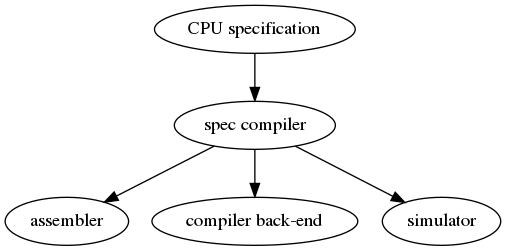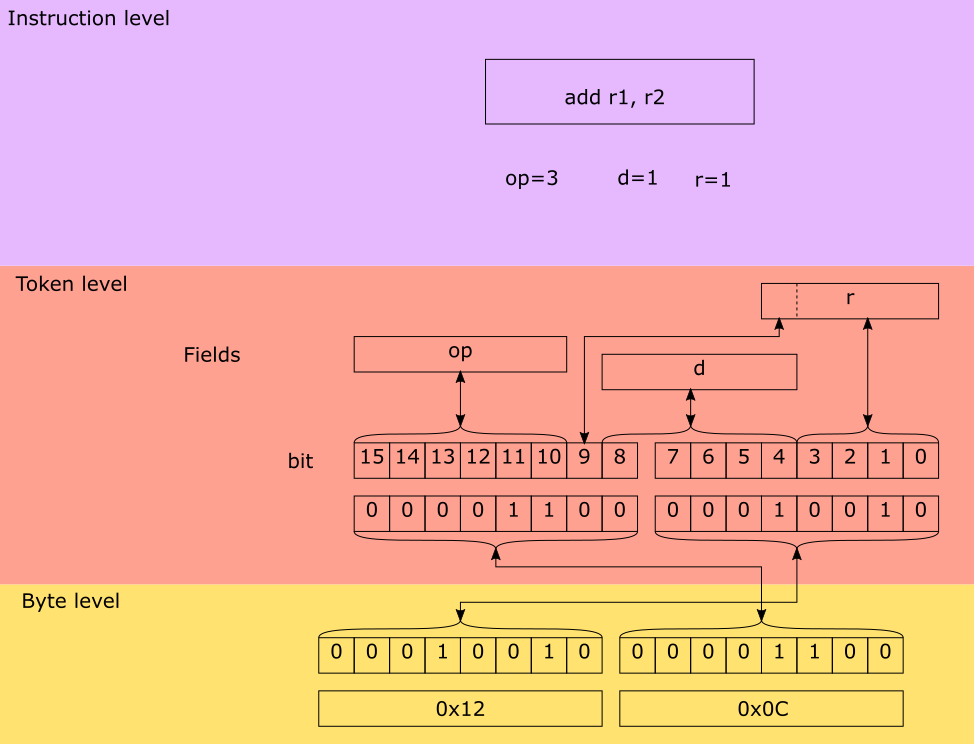# Specification languages¶

## Introduction¶

DRY

Do not repeat yourself (DRY). This is perhaps the most important idea to keep in mind when writing tools like assemblers, disassemblers, linkers, debuggers and compiler code generators. Writing these tools can be a repetitive and error prone task.

One way to achieve this is to to write a specification file for a specific processor and generate from this file the different tools. The goal of a machine description file is to describe a file and generate tools like assemblers, disassemblers, linkers, debuggers and simulators.## Design¶

The following information must be captured in the specification file:

• Assembly textual representation
• Binary representation
• Mapping from compiler back-end
• Effects of instruction (semantics)

The following image depicts the encoding and decoding of the AVR add instruction.The following code demonstrates how this instruction is described.

First the proper token is defined:

```from ppci.arch.token import Token, bit, bit_range, bit_concat

class AvrArithmaticToken(Token):
class Info:
size = 16

op = bit_range(10, 16)
r = bit_concat(bit(9), bit_range(0, 4))
d = bit_range(4, 9)
```

Then the instruction is defined, defining a syntax and the mapping of token fields to instruction parameters:

```from ppci.arch.avr.registers import AvrRegister
from ppci.arch.encoding import Instruction, Operand, Syntax

tokens = [AvrArithmaticToken]
rd = Operand('rd', AvrRegister, read=True, write=True)
rr = Operand('rr', AvrRegister, read=True)
syntax = Syntax(['add', ' ', rd, ',', ' ', rr])
patterns = {'op': 0b11, 'r': rr, 'd': rd}
```
```>>> from ppci.arch.avr import registers
>>> a1 = Add(registers.r1, registers.r2)
>>> str(a1)
>>> a1.encode()
b'\x12\x0c'
```

## Background¶

There are several existing languages to describe machines in a Domain Specific Language (DSL). Examples of these are:

• Tablegen (llvm)
• cgen (gnu)
• LISA (Aachen)
• nML (Berlin)
• SLED (Specifying representations of machine instructions (norman ramsey and Mary F. Fernandez))

http://www.cs.tufts.edu/~nr/toolkit/

Concepts to use in this language:

• Single stream of instructions
• State stored in memory
• Pipelining
• Instruction semantics

Optionally a description in terms of compiler code generation can be attached to this. But perhaps this clutters the description too much and we need to put it elsewhere.

The description language can help to expand these descriptions by expanding the permutations.

## Example specifications¶

For a complete overview of ADL (Architecture Description Language) see [overview].

### llvm¶

```def IMUL64rr : RI<0xAF, MRMSrcReg, (outs GR64:\$dst),
(ins GR64:\$src1, GR64:\$src2),
"imul{q}\t{\$src2, \$dst|\$dst, \$src2}",
[(set GR64:\$dst, EFLAGS,
(X86smul_flag GR64:\$src1, GR64:\$src2))],
IIC_IMUL64_RR>,
TB;
```

### LISA¶

```<insn> BC
{
<decode>
{
%ID: {0x7495, 0x0483}
%cond_code: { %OPCODE1 & 0x7F }
%dest_address: { %OPCODE2 }
}
<schedule>
{
BC1(PF, w:ebus_addr, w:pc) |
BC2(PF, w:pc), BC3(IF) |
BC4(ID) |
<if> (condition[cond_code])
{
BC5(AC) |
BC6(PF), BC7(ID), BC8(RE) |
BC9(EX)
}
<else>
{
k:NOP(IF), BC10(AC, w:pc) |
BC11(PF), BC12(ID), BC13(RE) |
k:NOP(ID), BC14(EX) |
k:NOP(ID), k:NOP(AC) |
k:NOP(AC), k:NOP(RE) |
k:NOP(RE), k:NOP(EX) |
k:NOP(EX)
}
}
<operate>
{
BC1.control: { ebus_addr = pc++; }
BC2.control: { ir = mem[ebus_addr]; pc++ }
BC10.control: { pc = (%OPCODE2) }
}
}
```

### SLED¶

```patterns
nullary is any of [ HALT NEG COM SHL SHR READ WRT NEWL NOOP TRA NOTR ],
which is op = 0 & adr = { 0 to 10 }
constructors
IMULb        Eaddr            is      (grp3.Eb;    Eaddr) & IMUL.AL.eAX
```

### nML¶

```type word = card(16)
type absa = card(9)
type disp = int(4)
type off = int(6)
mem PC[1,word]
mem R[16,word]
mem M[65536,word]
var L1[1,word]
var L2[1,word]
var L3[1,word]
mode register(i:card(4)) = R[i]
syntax = format(”R%s”, i)
image = format(”%4b”, i)
mode memory = ind | post | abs
mode ind(r:register, d:disp) = M[r+d]
update = {}
syntax = format(”@%s(%d)”, r.syntax, d)
image = format(”0%4b%4b0”, r.image, d)
mode post(r:register, d:disp) = M[r+d]
update = { r = r + 1; }
syntax = format(”@%s++(%d)”, r.syntax, d)
image = format(”0%4b%4b1”, r.image, d)
mode abs(a : absa) = M[a]
update = {}
syntax = format(”%d”, a)
image = format(”1%9b”, a)
op instruction( i : instr )
syntax = i.syntax
image = i.image
action = {
PC = PC + 1;
i.action;
}
op instr = move | alu | jump
op move(lore:card(1), r:register, m:memory)
syntax = format(”MOVE%d %s %s”, lore, r.syntax, m.syntax)
image = format(”0%1b%4b%10b”, lore, r.image, m.image)
action = {
if ( lore ) then r = m;
else m = r;
endif;
m.update;
}
op alu(s1:register, s2:register, d:reg, a:aluop)
syntax = format(”%s %s %s %s”, a.syntax, s1.syntax, s2.syntax, d.syntax)
image = format(”10%4b%4b%4b%2b”, s1.image, s2.image, d.image, a.image)
action = {
L1 = s1; L2 = s2; a.action; d = L3;
}
op jump(s1:register, s2:register, o:off)
syntax = format(”JUMP %s %s %d”, s1.syntax, s2.syntax, o)
image = format(”11%4b%4b%6b”, s1.image, s2.image, o)
action = {
if ( s1 >= S2 ) then PC = PC + o;
endif;
}
op aluop = and | add | sub | shift;
op and() syntax = ”and” image = ”00” action = { L3 = L1 & L2; }
op add() syntax = ”add” image = ”10” action = { L3 = L1 + L2; }
op sub() syntax = ”sub” image = ”01” action = { L3 = L1 - L2; }
```